## Questions

INSTRUCTIONS TO CANDIDATES• This paper consists of two sections: Section I and Section II.
• Answer ALL questions in section 1 and ONLY FIVE questions from section II
• All answers and workings must be written on the question paper in the spaces provided below each question.
• Show all the steps in your calculation, giving your answer at each stage in the spaces below each question.
• Non â Programmable silent electronic calculators and KNEC mathematical tables may be used, except where stated otherwise.

Section I (50 marks)
Answer all the questions in this section

1. A farmer wishes to enclose a rectangular nursery against a long straight wall. He has 40m
fencing wire. What is the largest area he can fence using the wire ? (3 marks)
2. Given that the vector a = 4 cm due North, and b= 5 cm North-East, find the magnitude of a + b. (3 marks)
3. What rate of compound interest per annum is equivalent to 2 percent compounded quarter yearly. (3 marks)
4. A point Q is 4 km due north of another point P. A man starts from P and walks in a direction 030Â°. Calculate how far he walks when he is
1. Equidistant from P and Q. (2 marks)
2. north-east of Q. (2 marks)
5. Six men take 56 hours to pack 2,240 parcels. Find the number of hours 5 men would take to pack 2,500 parcels if they worked at the same rate. (2 marks)
6. Given that x2 â 4x + y2 â 6y + 4 = 0 is an equation of a circle, determine the centre and radius of the circle. (3 marks)
7. Water flows from a circular pipe of diameter 3.5cm into a rectangular tank 66cm long, 35cm wide and 45cm high. If it takes 18 minutes to fill the tank completely, determine the rate of flow of the water in the pipe in cm/s? (3 marks)
8. Find the value of x if the determinant of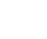= determinant of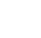. (3 marks)
9. Y varies partly as x and partly as the square of X. When X = 2, Y = 14 and when X = 4 Y = 44. Find Y when X = 6. (4 marks)
10. The chords PQ and ST meet at a point O outside the circle. Given that PQ = 3cm, QO = 5 cm and ST = 6 cm, calculate SO. (3 marks)
11. The tangent to the curve y = ax2 + bx + c is parallel to the line y - 4x=0 at the point where x = 2. If the curve has a minimum value of â3 where x = 1, find the values of a, b and c. (3 marks)
12. Show that (Cos x + Sin x) 2 + (Cos x â Sin x) 2 = 2. (3 marks)
13.
1. Expand  (3 + Â¹/âx)4 in ascending powers of x. (2 marks)
2. Use the first three terms of the expansion above to approximate the value of (3.025)4 to 3 decimal places. (2 marks)
14. A student recorded the length of a copper wire as 20.66 cm. If the actual length was 20.6 cm, calculate the fractional error committed. (3 marks)
15. The events A and B are mutually exclusive events. The probability of event A or B occurring is 9/20 and that of event A occurring is 1/5 . Find the probability of event B occurring. (2 marks)
16. The parabola y = x2 + 2 and y = 4x â x2 touch each other. Find the equation of the tangent at the point of contact. (4 marks)

SECTION II (50 MARKS)
Answer any five questions in this section1. Consider points A (50Â°N, 30Â°E) and B (50Â°N, 150Â°W) (Take Ï= 22â7) and radius of the earth R = 6370 km. Find:
1. The distance between A and B along a parallel of latitude in:
1. Kilometres (km) (3 marks)
2. Nautical miles (nm) (2 marks)
2. The shortest distance from A to B in nautical miles. (3 marks)
3. An aircraft takes 54 hours to fly between the two towns A and B along the great circle. Calculate its speed in knots correct to 2 significant figures. (2 marks)
2. ABCDE is a right pyramid on a horizontal square base of side 10 cm. The slant edges are all 8 cm long. Calculate;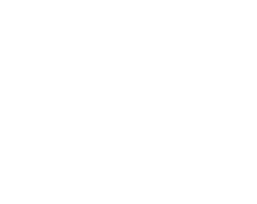1. The height of the pyramid (3 marks)
2. The angle between;
1. A slant face and the base (2 marks)
2. A slant edge and the base (2 marks)
3. The angle between the planes ABE and DCE (3 marks)
3.
1. ABCD is a quadrilateral with vertices as follows: A (3, 1), B (2, 4),C (4, 3) and D (5, 1)
1. A'B'C'D'is the image of ABCDunder a transformation whose matrix is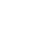. On the grid provided draw the quadrilateralABCD and its image A'B'C'D'. (3marks)
2. Describe fully the transformation that maps ABCD onto A'B'C'D'. (1mark)
2. A transformation represented by the matrix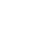maps A'B'C'D' onto A''B''C''D''. Plot A''B''C''D'' on the same grid. (3marks)
3. Determine a single matrix transformation that maps A''B''C''D'' onto ABCD. Describe this transformation fully. (3marks)
4. Two circles with centres O and Q and radii 8cm intersect at points A and B as shown below.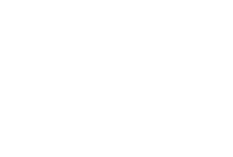Given that the distance between O and Q is 12cm and that the line AB meets OQ at X, find:
1. the length of the chord AB. (3marks)
2. the reflex angle AOB. (3marks)
3. the area of the shaded region. ( 4marks)
5. A bag contains 3 black balls and 6 white balls. If two balls are drawn from the bag one a time, find the:-
1. Probability of drawing two white balls
1. with replacement (2marks)
2. without replacement (2marks)
2. Probability of drawing a black ball and white ball.
1. with replacement (3marks)
2. without replacement (3marks)
6.
1. Using the trapezium rule, estimate the area enclosed by the curve y=x^2, the x-axis and the line x=5 and x=12 taking 7 ordinates. (3 marks)
2. Find the exact area. (3 marks)
3. Find the % error in using trapezium rule. (2 marks)
4. Estimate the area using mid-ordinate rule. (2 marks)
7.
1. The first term of an Arithmetic Progression (AP) is 2. The sum of the first 8 terms of the AP is 156.
1. Find the common difference of the AP (2marks)
2. Given that the sum of the first n terms of the AP is 416, find n. (2marks)
2. The 3rd, 5thand 8thterms of another AP from the first three terms of a Geometric progression (GP). If the common difference of the AP is 3, Find:
1. The first term of the GP (4marks)
2. The sum of the first 9 terms of the GP, to 4 significant figures. (2marks)
8. Three quantities P, Q and R and such that P varies directly as the square of Q and inversely as the square root of R.
1. Write an expression of P in terms of Q and R (1 mark)
2. Given that when P = 40, Q = 10 and R = 16. Find the value of P when Q = 7 and R = 64 (4 marks)
3. If Q is increased by 10% and R is decreased by 19%, find the percentage change in P. f(5 marks)

## Marking Scheme

1. 2x + y = 40
y- 40 - 2x
A= x (40 - 2x)
= 40x - 20x2
dA/dx = 40 - 4x = 0
x = 5, y = 30
A= 5 x 30 = 150

2. x2 = 42 + 52 - 2 x 4 x 5Cos45
x2 = 16 + 25 - 40Cos45

3. P(1 + r/100)1 = P(1 + 2/100)4
1 + r/100 = 1.024
r/100 = 1.024 - 1
r = 4.04
4.
1. Cos 300 = 4/x
x = 3.464

2. Sin 30 = x/4
x = 2

5. 56 x 6/5 x 2500/2240 = 75h

6. x - 4x + y2 - 6y = -4
(x - 2)2 + (y-3)2 = -4+4+9
(x-2)2 + (y-3)2=9
Centre (2,3)

7. V = 66 x 35 x 45 = 96.25
18 x 60
22/7 x 1.752 x V = 96.25
V =   96.25
9.625
= 10cm/s

8. x + 6 = 3x2 - 4
3x2 - x - 10 = 0
(3x + 5)(x - 2)
x = -5/3 = -12/3
x = 2
9. y = ax + bx2
14 = 2a + 4b
44 = 4a + 16b
a=3 and b=2
y= 3x6+2x62

10.  (6 + TO)TO = 8 x5
(TO)2 + 6TO - 40 = 0
(TO + 10)(TO - 4)= 0
TO = 4
SO=10

11.  dy/dx = 2ax + 4
2a(2) + b = 4
4a + b = 4
2a + b = 0
a= 2
b= -4
-3 = 2 - 4 + c
c= -1

12. Cos 2x + 2CosSinx + Sin2x + Cos2x - 2Cos XSin 2Y
= 2Cos2x + 2Sin2x
=2(Cos2x + Sin2 x)
=2(1)= 2

13. (3 + Â¹/âx)4
= 1.34 + 4.33(Â¹/âx) + 6.32(Â¹/âx)2 + 4.3(Â¹/âx)3 + (Â¹/âx)4
= 81 + 27/2 x + 27/32x2 + 3/128x3 + xâ´/4096
= (3.025)4
x = 0.2
3.0254 - 81 + 27(0.2) + 27(0.2)2 + 3(0.2)20.2â´
2              32             128       4096
= 83.72

14. Error 20.66 - 20.6 = 0.6
Fractional error
0.06    =     6
20.6          2060
3/1030

15. P(B) + 1/5 = 9/20
P(B) = 9/20 - 1/5
= 1/4

16. x2 + 2 = 4x - x2
2x2 - 4x + 2 = 0
x2 - 2x + 1 = 0
(x-2)(x-2)= 0
x = 2
y= 22 + 2 = 6
dy/dx = 2x
M = 2x2= 4
Equation â
y-6  = 4
x-2
y= 4x + 2
17.
1.
1. 180/360 x 2 x 22/7 x 6370 Cos 50 = 12868.6
2. 180 x 60 Cos 50 = 6942.106125nm

2. Shortest distance
= 80 x 60 = 4800nm

3. 4800
54
= 88.888 â 89 knots

1.  Diagonal = â200 = 14.142
h = â82 - 7.0712
= â14 = 3.742
2.
1. tan Î¸ = 3.742/5
Î¸ = 36.81

2. Sin Î¸ = 3.742/8
Î¸ = 27.89

3. Tan Î¸ = 5/3.742
Î¸ = 53.19
Angle - 53.19 x 2
= 106.38
18.
1.
1.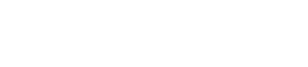A'(-1, 3) B' (-4, 2) C' (-3, 4)  D'(-1, 5)

2. 1/4 turn about the origin

2.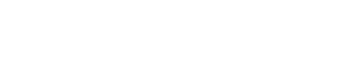A'' (-1-3) B' (-4, 2) C''(-3, -4) D''(-1, -5)

3.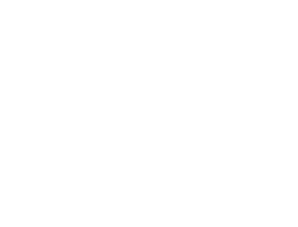Reflection on the line y= -x
19.
1. AB/2 = â(82 - 62)
Ab/2 = 5.2915
Ab = 5.295 x 2
= 10.58

2. Cos Î¸ = 3/4 = 0.75
Î¸ = 41.41
reflex angle = 41.41 x 2 = 82.82
360 = 82.82
= 277.18Â°

3. 82.82 x 31.42 x 64 - 1/2 x 64.55 x 82.82
360
46.26 - 31.75= 14.51
= 31.75 x 2 - 14.51 x 2
= 63.5 - 29.02
=34.48
20.
1.
1. 6/9 x 6/9 = 36/81
2. P(ww)= 6/9 x 5/8 = 30/72
2.
1. P(wb or bw)
= (6/9 x 3/9) + (3/9 x 6/9) = 36/81
2. q(wb or bw)
=(6/9 x 3/8) + (3/9 x 6/9)= 36/72
21.
1.
 x 5 6 7 8 9 10 11 12 y 25 36 49 64 81 100 121 144
A = 1/2 x 1(25 + 144)(36 + 49 + 64 + 81 + 100 + 121)
= 1/2 (169 + 2 x 451)= 535.5

2. â«12x2dx = [xÂ³/3]2
1728  125
3            3
= 534 1/3

3. 535 Â¹/â - 534Â¹/â  x 100%
534Â¹/â
= 0.2183
4.
 x 5.5 6.5 7.5 8.5 9.5 10.5 11.5 y 30.25 42.25 56.25 72.25 90.25 110.25 132.25

A = 1(30.25 + 42.25 + 56.25 + 72.25 + 90.25 + 110.25 + 132.25) = 533.75
22.
1. P = KQ2
âR

2. 40 = K (10)2
â6
K = 40 x 4
100
= 1.6

3. New P = K (1.21)Q2
0.9âR
= 1.3444KQ2
âR
Change = 1.3444KQ2  KQ2
âR              âR
%age change = 0.3444    KQ  x 100
KQ2/R    âR
= 34.44
23.
1. P= kQ2
âR

2. P=40 , q=10 , r = 16
P= kQ2
âR
40 = 100k
4
160 = 100k
1.6 = k
49 x 1.6 = 9.8
8
3. Q â 10
1.1Q â 11
R â 16
0.81R â12.96

1.6 x 121 = 16 x 121 x 10  = 4 x 4 x 11 x 11 x 10
3.6             36 x 10              4 x 9 x 10
4 x 121 = 484 = 53 7/9
9           9
40 â 100
484/9 â?
484/9 x 1/40 x 100 = 134 4/9
percentage incease = 34 4/9